欢迎访问我的网站，希望内容对您有用，感兴趣的可以加入免费知识星球。

# 一款Python高性能数据处理工具

2个月前 (10-02) 289次浏览

## 简介

`Polars` 是一个开源的 `Python` 库，是一个高性能数据处理库，提供了类似于 `Pandas``API` 接口。它在处理大规模数据时表现出色，能够快速执行各种数据操作，如筛选、转换、连接等。

## 安装

``pip install polars pyarrow``

## 基本使用

### 创建和加载数据

`Polars` 中，可以使用 `polars.DataFrame` 对象来表示数据。可以通过多种方式创建和加载数据，如手动创建、从 `CSV` 文件加载、从 `Pandas DataFrame` 转换等。以下是一些常用的示例代码

``````import polars as pl
import pandas as pd

# 创建DataFrame
df = pl.DataFrame({
'name': ['Alice', 'Bob', 'Charlie'],
'age': [25, 30, 35]
})

# 从CSV文件加载数据

# 从Pandas DataFrame转换
pandas_df = pd.DataFrame({'name': ['Alice', 'Bob', 'Charlie'], 'age': [25, 30, 35]})
df = pl.from_pandas(pandas_df)
print(df)``````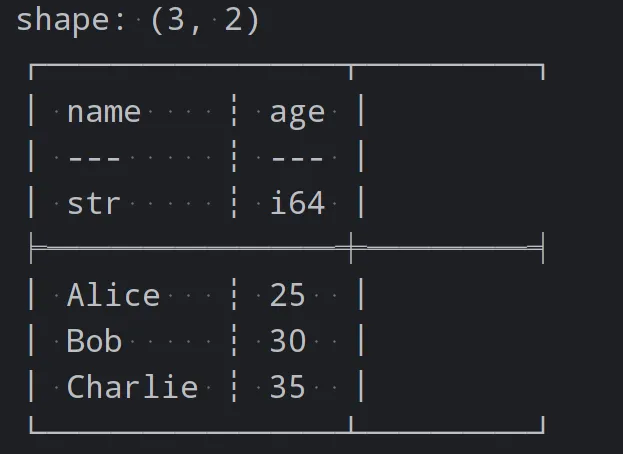### 数据筛选和过滤

`Polars` 提供了灵活的筛选和过滤功能，可以根据条件选择特定的行或列。以下是一些示例代码

``````# 根据条件筛选行
filtered_df = df.filter(pl.col("age") > 30)

# 根据条件筛选列
selected_df = df[['name', 'age']]
print(selected_df)

# 使用多个条件筛选
filtered_df = df.filter((pl.col("age") > 30) & (pl.col("name") == "Bob"))
print(filtered_df)``````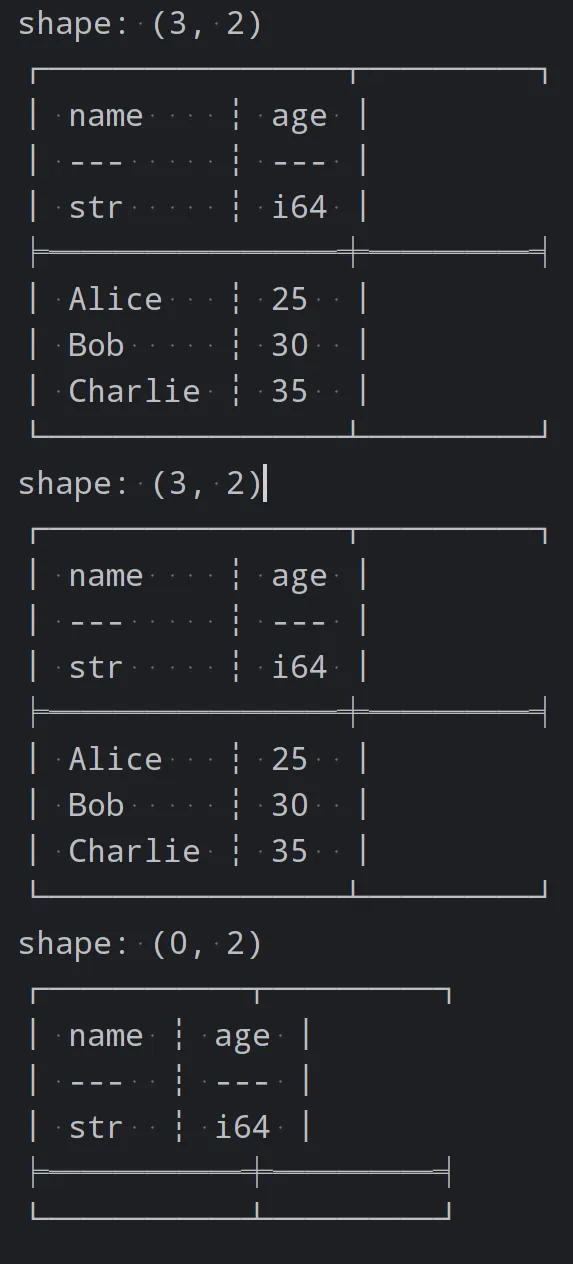### 数据转换

`Polars` 提供了丰富的数据转换和操作功能，如添加新列、重命名列、排序等。以下是一些示例代码

``````# 添加新列height
df = df.with_columns(pl.Series('height', [160, 170, 180]))

# 重命名age列
df = df.with_columns(pl.col('age').alias('years'))

# 按列进行排序
sorted_df = df.sort('age')
print(sorted_df)``````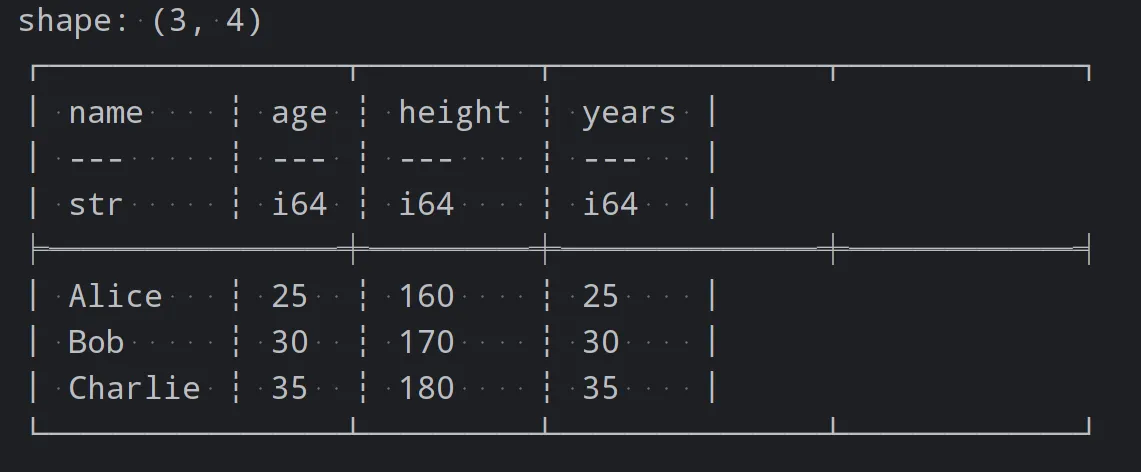### 数据聚合和分组

`Polars` 支持灵活的数据聚合和分组操作，可以根据指定的列对数据进行分组，并进行各种聚合操作，如求和、计数、平均值等。以下是一些示例代码

``````import polars as pl

# 创建DataFrame
df = pl.DataFrame({
'name': ['fish', 'meat', 'fish', 'meat'],
'price': [25, 30, 35, 40]
})

# 按name列分组，并计算每个类别的平均售价
grouped_df = df.group_by('name').agg(pl.col('price').mean())
print(grouped_df)``````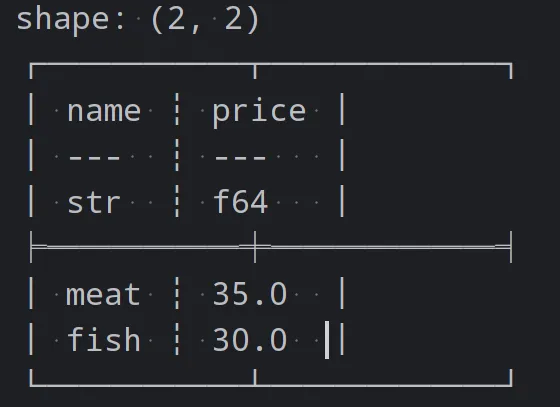### 数据连接

`Polars` 提供了多种数据连接的方法，如合并两个 `DataFrame`、连接两个 `DataFrame` 等。以下是一个示例代码

``````import polars as pl

# 创建DataFrame
df = pl.DataFrame({
'name': ['fish', 'meat'],
'price': [25, 30]
})

df1 = pl.DataFrame({
'name': ['beef', 'vegetable'],
'price': [65, 10]
})

# 连接两个DataFrame
joined_df = df.join(df1, on='name', how="outer")
print(joined_df)``````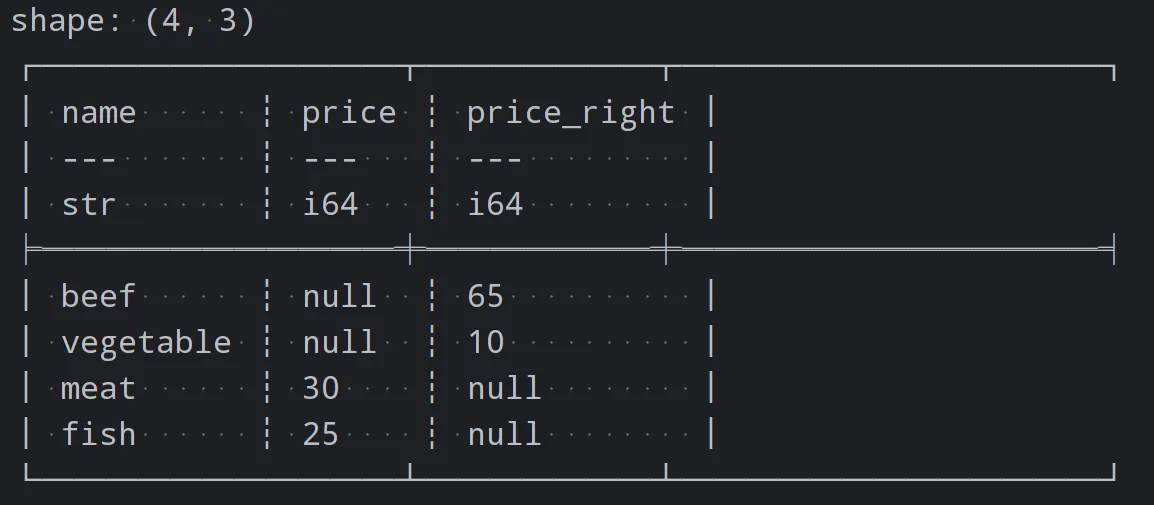### 自定义函数和映射

`Polars` 中，可以使用自定义函数和映射来对数据进行复杂的转换和操作。以下是一个示例代码

``````import polars as pl

# 创建DataFrame
df = pl.DataFrame({
'name': ['Alice', 'Bob', 'Charlie'],
'age': [25, 30, 35]
})

# 定义自定义函数
def double_age(age):
return age * 2

# 应用自定义函数
df = df.with_columns(pl.col('age').map_batches(double_age).alias("double_age"))
print(df)``````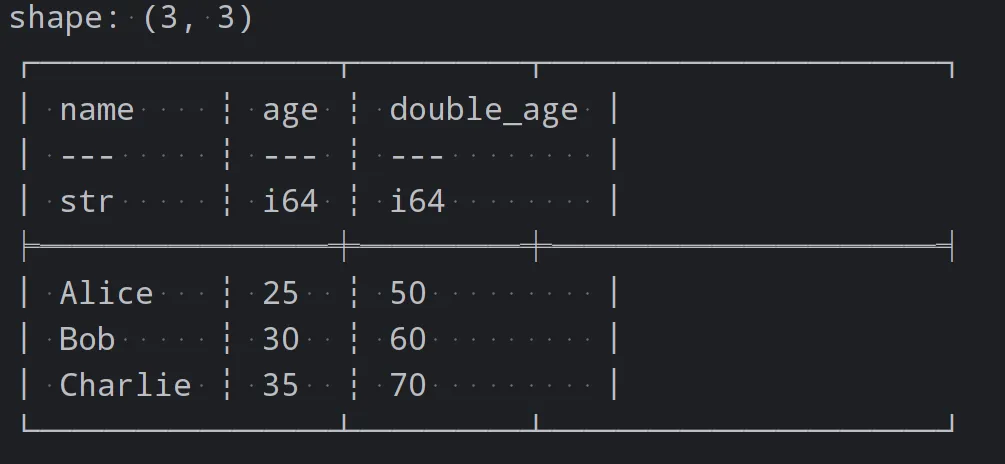/* */